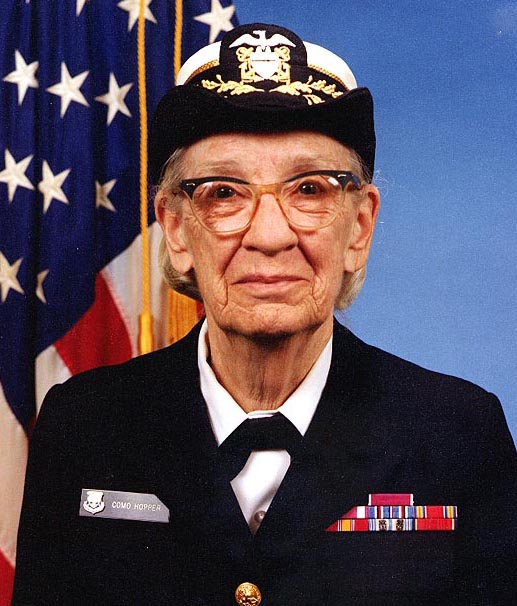Page History

Key

• This line was removed.
• Formatting was changed.
Comment: 修正翻译文档

Panel
borderColor #00FFFF dashed

 校对：Ngxin

Inception-v3 使用2012年的数据对v3 对ImageNet大型视觉识别挑战进行了培训。这是计算机视觉中的一项标准任务，其中模型尝试将整个图像分为大型视觉识别挑战在2012年采用的数据集进行训练。这是计算机视觉中的一项标准任务，其中模型尝试将整个图像分为1000个类，如“斑马”，“达尔马提亚”和“洗碗机”。例如，以下是，如“Zebra”，“Dalmatian”和“Dishwasher”。例如，以下是AlexNet对一些图像进行分类的结果：使用Python API

`classify_image.py`从tensorflow在程序第一次运行时从tensorflow.org 第一次运行程序时下载训练有素的模型。您的硬盘上可能需要大约200M的可用空间。org下载训练模型。您的硬盘上可能需要大约200M的可用空间。

Code Block
language py
```cd models/tutorials/image/imagenet
python classify_image.py```Code Block
language py
```giant panda, panda, panda bear, coon bear, Ailuropoda melanoleuca (score = 0.88493)
indri, indris, Indri indri, Indri brevicaudatus (score = 0.00878)
lesser panda, red panda, panda, bear cat, cat bear, Ailurus fulgens (score = 0.00317)
custard apple (score = 0.00149)
earthstar (score = 0.00127)```

使用C ++ API

Code Block
language py
```curl -L "https://storage.googleapis.com/download.tensorflow.org/models/inception_v3_2016_08_28_frozen.pb.tar.gz" |
tar -C tensorflow/examples/label_image/data -xz ```
`接下来，我们需要编译包含加载和运行图形代码的C接下来，我们需要编译包含加载和运行图代码的C ++二进制文件。如果您按照 说明下载适用 于您的平台的TensorFlow的源代码安装，您应该能够通过从您的shell终端运行此命令来构建该示例：二进制文件。如果您已经在开发平台按照 源代码安装TensorFlow的说明安装好tensorflow，您应该能够通过从您的shell终端运行此命令来构建该示例：`
Code Block
language py
`bazel build tensorflow/examples/label_image/...`
`那应该创建一个二进制可执行文件，然后你可以这样运行：创建一个二进制可执行文件，然后你可以这样运行：`
Code Block
language py
`bazel-bin/tensorflow/examples/label_image/label_image `
`这使用框架附带的默认示例图像，并应输出类似于此的内容：`
Code Block
language py
```I tensorflow/examples/label_image/main.cc:206] military uniform (653): 0.834306
I tensorflow/examples/label_image/main.cc:206] mortarboard (668): 0.0218692
I tensorflow/examples/label_image/main.cc:206] academic gown (401): 0.0103579
I tensorflow/examples/label_image/main.cc:206] pickelhaube (716): 0.00800814
I tensorflow/examples/label_image/main.cc:206] bulletproof vest (466): 0.00535088```
`在这种情况下，我们正在使用海军上将魔法师的默认图像 ，您可以看到网络正确识别她穿着军装，得分高达0在这种情况下，我们正在使用默认的Admiral Grace Hopper图像 ，您可以看到网络正确识别她穿着军装，得分高达0.8。`Code Block
language py
`bazel-bin/tensorflow/examples/label_image/label_image --image=my_image.png`
`如果您查看`tensorflow/examples/label_image/main.cc` 文件内容，可以了解它的工作原理。我们希望这段代码可以帮助您将TensorFlow集成到您自己的应用程序中，因此我们将逐步介绍主要功能： 文件内容，可以了解它的工作原理。我们希望这段代码可以帮助您将TensorFlow集成到您自己`

`的应用程序中，因此我们将逐步介绍主要功能：`

Code Block
language py
```// Given an image file name, read in the data, try to decode it as an image,
// resize it to the requested size, and then scale the values as desired.
Status ReadTensorFromImageFile(string file_name, const int input_height,
const int input_width, const float input_mean,
const float input_std,
std::vector<Tensor>* out_tensors) {
tensorflow::GraphDefBuilder b;```
`我们首先创建一个`GraphDefBuilder`可以用来指定运行或加载的模型的对象。`
Code Block
language py
```  string input_name = "file_reader";
string output_name = "normalized";
b.opts().WithName(input_name));```
`然后，我们开始创建我们要运行的小型模型的节点，以加载，调整大小和缩放像素值，以获得主模型期望作为其输入的结果。我们创建的第一个节点只是一个`Const`op，它包含我们要加载的图像的文件名的张量。那就是作为第一个输入`ReadFile`。您可能会注意到我们然后，我们开始创建我们要运行的小型模型的节点，通过加载、调整大小和缩放像素值，以获得主模型期望作为输入的结果。我们创建的第一个节点只是一个`Const`操作，它包含我们要加载的图片文件名的张量。把第一个输入传给`ReadFile操作`。您可能会注意到我们把`b.opts()`作为最后一个参数传递给所有op创建函数。该参数确保将节点添加到模型定义中`GraphDefBuilder`。我们也打电话给`ReadFile` 运营商。这给了一个节点的名字，这并不是绝对必要的，因为如果你不这样做，就会分配一个自动的名字，但这样会使调试变得更容易一些。作为最后一个参数传递给所有操作创建的函数。该参数确保在 `GraphDefBuilder` 中，将节点添加到模型定义中。我们通过给 `WithName()` 传入  `b.opts()` 命名`ReadFile` 操作。 这并不是必要的，因为如果你不这样做，就会分配一个自动的名字，但这样做会使调试变得更容易一些。 `
Code Block
language py
```  // Now try to figure out what kind of file it is and decode it.
const int wanted_channels = 3;
if (tensorflow::StringPiece(file_name).ends_with(".png")) {
} else {
// Assume if it's not a PNG then it must be a JPEG.
}
// Now cast the image data to float so we can do normal math on it.
tensorflow::Node* float_caster = tensorflow::ops::Cast(
// The convention for image ops in TensorFlow is that all images are expected
// to be in batches, so that they're four-dimensional arrays with indices of
// [batch, height, width, channel]. Because we only have a single image, we
tensorflow::Node* dims_expander = tensorflow::ops::ExpandDims(
float_caster, tensorflow::ops::Const(0, b.opts()), b.opts());
// Bilinearly resize the image to fit the required dimensions.
tensorflow::Node* resized = tensorflow::ops::ResizeBilinear(
dims_expander, tensorflow::ops::Const({input_height, input_width},
b.opts().WithName("size")),
b.opts());
// Subtract the mean and divide by the scale.
tensorflow::ops::Div(
tensorflow::ops::Sub(
resized, tensorflow::ops::Const({input_mean}, b.opts()), b.opts()),
tensorflow::ops::Const({input_std}, b.opts()),
b.opts().WithName(output_name));```
`然后，我们继续添加更多的节点，将文件数据解码为图像，将整数转换为浮点值，调整其大小，然后最终对像素值执行减法和除法运算。`
Code Block
language py
```  // This runs the GraphDef network definition that we've just constructed, and
// returns the results in the output tensor.
tensorflow::GraphDef graph;
TF_RETURN_IF_ERROR(b.ToGraphDef(&graph)); ```
`最后，我们有一个存储在b变量中的模型定义，我们将其转换成具有该`ToGraphDef()`函数的完整图形定义。`
Code Block
language py
```  std::unique_ptr<tensorflow::Session> session(
tensorflow::NewSession(tensorflow::SessionOptions()));
TF_RETURN_IF_ERROR(session->Create(graph));
TF_RETURN_IF_ERROR(session->Run({}, {output_name}, {}, out_tensors));
return Status::OK();```
`然后我们创建一个`tf.Session` 对象，它是实际运行图形的接口，并运行它，指定要获取输出的节点以及输出数据的位置。`

Code Block
language py
```// Reads a model graph definition from disk, and creates a session object you
// can use to run it.
std::unique_ptr<tensorflow::Session>* session) {
tensorflow::GraphDef graph_def;
return tensorflow::errors::NotFound("Failed to load compute graph at '",
graph_file_name, "'");
}```
`如果您浏览了图像加载代码，则很多术语应该看起来很熟悉。而不是使用a 如果您浏览了图像加载代码，那么很多术语应该看起来很熟悉。而不是使用`GraphDefBuilder`来生成一个`GraphDef`对象，我们加载一个直接包含的protobuf文件对象，我们加载一个包含`GraphDef`的protobuf文件。`
Code Block
language py
```  session->reset(tensorflow::NewSession(tensorflow::SessionOptions()));
Status session_create_status = (*session)->Create(graph_def);
if (!session_create_status.ok()) {
return session_create_status;
}
return Status::OK();
}```
`然后我们创建一个Session对象，然后我们从`GraphDef` 创建一个Session对象，并将其传递给调用者，以便以后可以运行它。`

`GetTopLabels()`功能非常像图像加载，除了在这种情况下，我们要获取运行主图的结果，并将其转换为最高评分标签的排序列表。就像图像加载器一样，它创建一个 函数非常像图像加载，除了我们要获取运行主图的结果，并将其转换为最高评分标签的排序列表。就像图像加载器一样，它创建一个 `GraphDefBuilder`，添加了几个节点，然后运行短图来获得一对输出张量。在这种情况下，它们表示最高结果的排序分数和索引位置。

Code Block
language py
```// Analyzes the output of the Inception graph to retrieve the highest scores and
// their positions in the tensor, which correspond to categories.
Status GetTopLabels(const std::vector<Tensor>& outputs, int how_many_labels,
Tensor* indices, Tensor* scores) {
tensorflow::GraphDefBuilder b;
string output_name = "top_k";
tensorflow::ops::TopK(tensorflow::ops::Const(outputs, b.opts()),
how_many_labels, b.opts().WithName(output_name));
// This runs the GraphDef network definition that we've just constructed, and
// returns the results in the output tensors.
tensorflow::GraphDef graph;
TF_RETURN_IF_ERROR(b.ToGraphDef(&graph));
std::unique_ptr<tensorflow::Session> session(
tensorflow::NewSession(tensorflow::SessionOptions()));
TF_RETURN_IF_ERROR(session->Create(graph));
// The TopK node returns two outputs, the scores and their original indices,
// so we have to append :0 and :1 to specify them both.
std::vector<Tensor> out_tensors;
TF_RETURN_IF_ERROR(session->Run({}, {output_name + ":0", output_name + ":1"},
{}, &out_tensors));
*scores = out_tensors;
*indices = out_tensors;
return Status::OK();```
`该`PrintTopLabels()`功能采用这些排序结果，并以友好的方式打印出来。该函数接受这些排序结果，并以用户友好的方式打印出来。`与``CheckTopLabel()`功能非常相似，但只是确保顶级标签是我们期望的标签，用于调试目的。函数非常相似，但只是确保顶级标签是我们期望的标签，以便用于调试。最后，`main()``
将所有这些电话联系在一起。
` 将所有函数调用联系在一起。`
Code Block
language py
```int main(int argc, char* argv[]) {
// We need to call this to set up global state for TensorFlow.
tensorflow::port::InitMain(argv, &argc, &argv);
Status s = tensorflow::ParseCommandLineFlags(&argc, argv);
if (!s.ok()) {
LOG(ERROR) << "Error parsing command line flags: " << s.ToString();
return -1;
}

// First we load and initialize the model.
std::unique_ptr<tensorflow::Session> session;
string graph_path = tensorflow::io::JoinPath(FLAGS_root_dir, FLAGS_graph);
return -1;
}```
`我们加载主图。`
Code Block
language py
```  // Get the image from disk as a float array of numbers, resized and normalized
// to the specifications the main graph expects.
std::vector<Tensor> resized_tensors;
string image_path = tensorflow::io::JoinPath(FLAGS_root_dir, FLAGS_image);
image_path, FLAGS_input_height, FLAGS_input_width, FLAGS_input_mean,
FLAGS_input_std, &resized_tensors);
return -1;
}
const Tensor& resized_tensor = resized_tensors;```
`加载，调整大小并处理输入图像。`
Code Block
language py
```  // Actually run the image through the model.
std::vector<Tensor> outputs;
Status run_status = session->Run({ {FLAGS_input_layer, resized_tensor}},
{FLAGS_output_layer}, {}, &outputs);
if (!run_status.ok()) {
LOG(ERROR) << "Running model failed: " << run_status;
return -1;
}```
`在这里，我们运行加载的图形作为输入图像。`
Code Block
language py
```  // This is for automated testing to make sure we get the expected result with
// the default settings. We know that label 866 (military uniform) should be
// the top label for the Admiral Hopper image.
if (FLAGS_self_test) {
bool expected_matches;
Status check_status = CheckTopLabel(outputs, 866, &expected_matches);
if (!check_status.ok()) {
LOG(ERROR) << "Running check failed: " << check_status;
return -1;
}
if (!expected_matches) {
LOG(ERROR) << "Self-test failed!";
return -1;
}
} ```
`为了测试的目的，我们可以检查以确保我们在这里得到我们预期的输出。为了测试，我们可以检查以确保我们在这里得到我们预期的输出。`
Code Block
language py
```  // Do something interesting with the results we've generated.
Status print_status = PrintTopLabels(outputs, FLAGS_labels);```
`最后我们打印出我们发现的标签。最后我们打印出标签。`
Code Block
language py
```  if (!print_status.ok()) {
LOG(ERROR) << "Running print failed: " << print_status;
return -1;
} ```
`这里的错误处理是使用TensorFlow的`Status` 对象，这是非常方便的，因为它可以让您知道`ok()`检查器是否发生任何错误，然后可以打印出来，提供可读的错误消息。检查器是否发生任何错误，然后可以打印出来，`

`使错误消息更具可读性。`

EXERCISE：迁移学习是一个想法，如果你知道如何很好的解决一个任务，你应该能够转移一些理解来解决相关的问题。迁移学习的一种方法是去除网络的最后一个分类层，并提取next-to-last layer of the CNN，在这种情况下为2048维向量。 在 how-to section中有一个关于这样操作的指导。